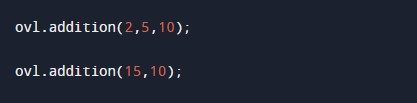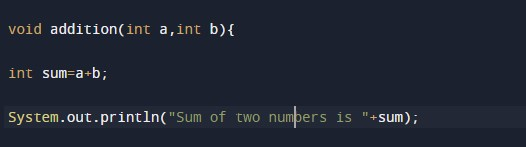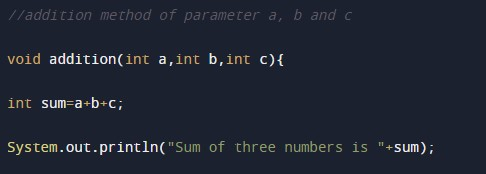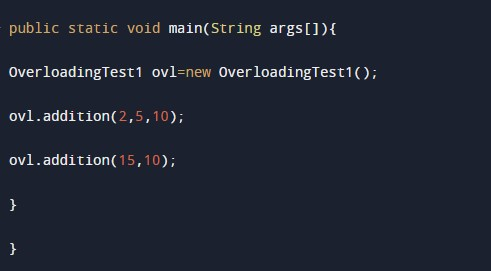In this tutorial you will learn about the Java Program to perform Arithmetic Operation using Method Overloading and its application with practical example.

In this tutorial, we will learn to create a Java program that will perform Arithmetic operations using Method Overloading in Java programming.

## Prerequisites

Before starting with this tutorial, we assume that you are the best aware of the following Java programming topics:

• Operators in Java Programming.
• Basic Input and Output function in Java Programming.
• Basic Java Programming.
• Arithmetic operations in java programming.

## Program to Description-:-

In today’s tutorial, we will create a program that will perform Arithmetic operations on the two numbers using the concepts method overloading. First, we will take input numbers from the user. We will use the arithmetic operators, in java, for calculations. Then we will print the output of different overloaded functions to the user. First, an addition function goes under the execution, then the overloaded method goes under execution.

With the help of this program, we can take input and perform the arithmetic operations.

## Output:-In the above program, we have first initialized the required variable.• a= it will hold the number value for the program.
• b = it will hold the number value for the program.
• sum = it will hold the sum value of the numbers.